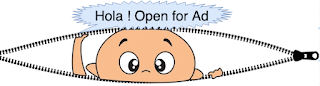## Latest Posts

In inorder traversal, left subtree is processed first followed by current node and then right subtree. In recursive version of inorder traversal stack(LIFO data structure) maintains references of left and right child.
In iterative version of inorder traversal we requires an external stack where references of left and right child is stored so that after visiting left subtree followed by current node, we can process right subtree. Here, with respect to each node, all left subtree is pushed into stack and process each node in loop. Loop is terminated once stack is empty.Refer following algorithm and sample code.
Algorithm:-
1. Insert root into stack, if root is not null.
2. Inside loop, do check for empty stack and terminate loop, if stack is empty.
1. Traverse given tree starting from current root until reaches leaf node, push all intermediate left subtree in stack.
2. pop elements from stack and process it
3. If right child of popped node exist then push right child in stack and right child becomes current root.
4. Repeat steps 1 to 3 until stack is empty.
Time and space complexity :- Time complexity is order of O(n) and space complexity is O(n).
Refer following binary tree for inorder traversal:-
Inorder traversal output should be :- 56 11 78 23 43 12 12 98 18
Java code Iterative inorder traversal
```public String iterativeInorderTraversal(Node root) {
/* java.util.Stack */
Stack<Node> stack = new Stack<>();
StringBuffer sbf = new StringBuffer();
if (root != null) {
stack.push(root);
while (true) {
/* push all left subtree in stack */
while (root != null) {
Node lc = root.leftChild;
/* If left subtree exist, push into stack */
if (lc != null)
stack.push(lc);
/* left child becomes root */
root = lc;
}// end inner while loop.

/*
* Check for outer loop termination, if stack is empty break
* loop, Otherwise pop and process element from stack
*/
if (stack.isEmpty()) {
break;
} else {
root = stack.pop();
/* process root data */
sbf.append(root.getData() + " ");

Node rc = root.getRightChild();
/* If right child exist, push into stack */
if (rc != null) {
stack.push(rc);
}
/* Now right child becomes root */
root = rc;
}
}
}
return sbf.toString();
}```

#### Complete sample program non-recursive inorder traversal of binary tree in Java

```package com.devinline.trees;

import java.util.Stack;

public class IterativeInorderTraversal {

public String iterativeInorderTraversal(Node root) {
/* java.util.Stack */
Stack<node> stack = new Stack&lt;&gt;();
StringBuffer sbf = new StringBuffer();
if (root != null) {
stack.push(root);
while (true) {
/* push all left subtree in stack */
while (root != null) {
Node lc = root.leftChild;
/* If left subtree exist, push into stack */
if (lc != null)
stack.push(lc);
/* left child becomes root */
root = lc;
}// end inner while loop.

/*
* Check for outer loop termination, if stack is empty break
* loop, Otherwise pop and process element from stack
*/
if (stack.isEmpty()) {
break;
} else {
root = stack.pop();
/* process root data */
sbf.append(root.getData() + " ");

Node rc = root.getRightChild();
/* If right child exist, push into stack */
if (rc != null) {
stack.push(rc);
}
/* Now right child becomes root */
root = rc;
}
}
}
return sbf.toString();
}

public static void main(String[] args) {
IterativeInorderTraversal itrInObj = new IterativeInorderTraversal();
Node root = itrInObj.new BinaryTree().createTree();
String nodesStr = itrInObj.iterativeInorderTraversal(root);
System.out.println("Iterative inorder traversal:-  " + nodesStr);
}

class BinaryTree {
Node root;

public BinaryTree() {
root = null;
}

public Node createTree() {
if (root == null) {
root = new Node(12);
}
root.setLeftChild(new Node(23));
root.setRightChild(new Node(18));
root.getLeftChild().setLeftChild(new Node(11));
root.getLeftChild().setRightChild(new Node(43));
root.getRightChild().setLeftChild(new Node(12));
root.getLeftChild().getLeftChild().setLeftChild(new Node(56));
root.getLeftChild().getLeftChild().setRightChild(new Node(78));
root.getRightChild().getLeftChild().setRightChild(new Node(98));
return root;
}
}

class Node {

private int data;
private Node leftChild;
private Node rightChild;

public Node(int data) {
this.data = data;
leftChild = null;
rightChild = null;
}

public int getData() {
return data;
}

public void setData(int data) {
this.data = data;
}

public Node getLeftChild() {
return leftChild;
}

public void setLeftChild(Node leftChild) {
this.leftChild = leftChild;
}

public Node getRightChild() {
return rightChild;
}

public void setRightChild(Node rightChild) {
this.rightChild = rightChild;
}
}

}
```
=========Sample output=============
Iterative inorder traversal:- 56 11 78 23 43 12 12 98 18
=================================

#### 1 comment:

1.